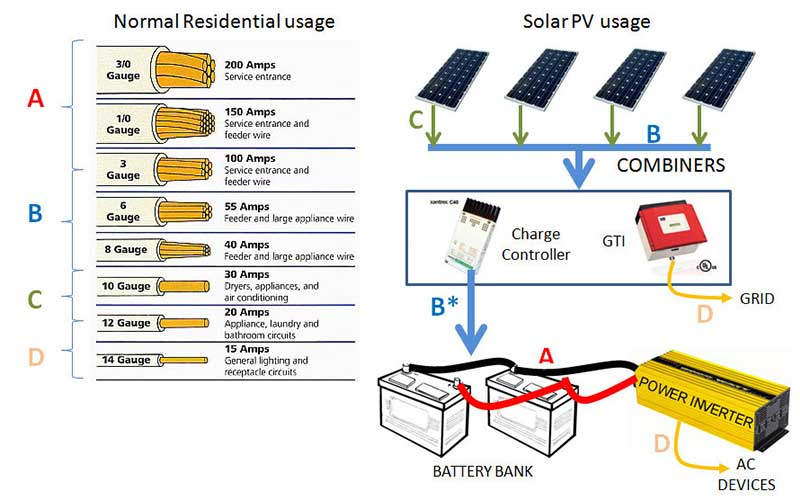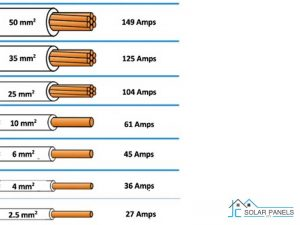# Calculating a solar system to meet your needs

### Flow of electricity

Electricity  is a source of energy that is transferred through conductors or wires. These are metal elements that transmit electrical force over a specific distance. To provide electricity to an appliance from one point to the point of the appliance, we make use of wires to connect the electricity to the appliances. A wire is a conductor inside an isolator. Therefore, the electricity can flow inside the wire but it cannot escape due to the insulating sheath around the wire. It can be compared to a water pipe; water can travel freely through the pipe but it cannot get out through the pipe except for by the opening end. Electricity in wires works in the same concept.  Therefore, if the water or electricity is trapped inside a pipe or wire, it can travel over a distance to reach its end destination.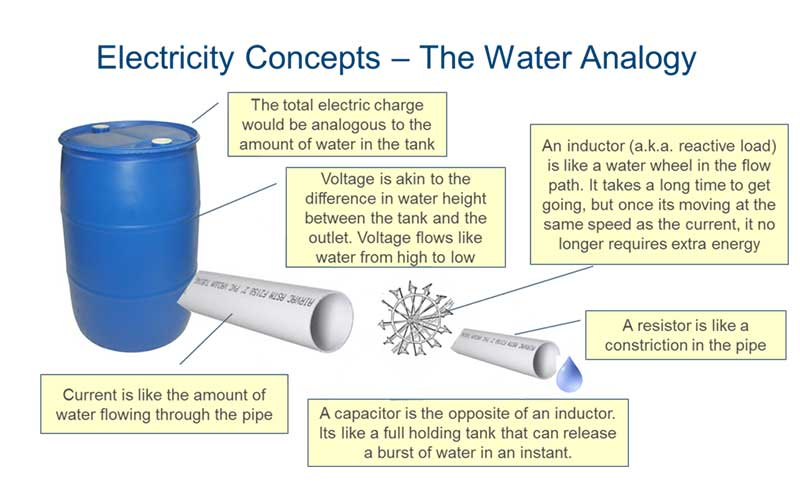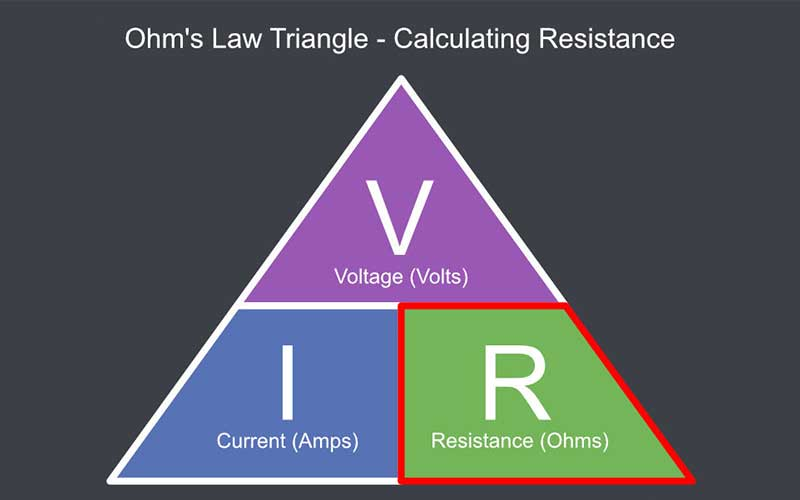### How is electricity measured?

To measure electricity, we have to use Volts, Amps, and Watts to calculate the amount of electrical force being transmitted by wire or conductor. The force of the electricity moving through the wire can be measured by Volts. Amps are measured to determine how much electrical energy is being transmitted through the conductor.

### What is Voltage

If we go back to our water pipe example, if the water inside the water pipe can be compared to electricity in a conductor, the pressure of the water inside the pipe can be compared to the voltage (pressure) of the electricity inside the conductor. The voltage or electrical pressure is constant and always available, even if the energy is not flowing. Therefore, Volts can be described as the potential of energy and it is always ready to be used. BY calculating your voltage, you can easily determine the appliances which you can connect to your electrical system. In other words, if you have an electrical device that operates on 12 volts, you can connect a 12 Volt battery to it to provide electricity. The same principle is true for appliances which required 240 Volts if you have a 240 Voltage outlet, you can connect that appliance to the 240 Voltage outlet for power. Voltage is a great way to determine which devices would be compatible with one another.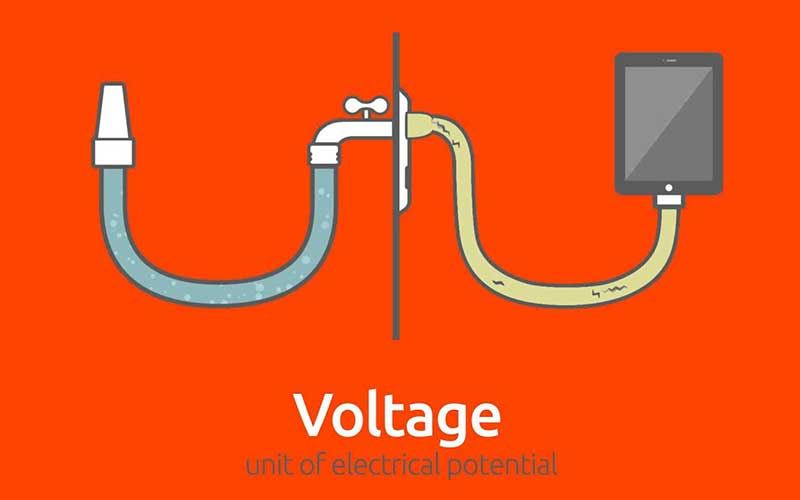### What is Amperage

Amperage is basically the amount of electricity that is being transmitted over electrical wires. Therefore, depending on a specific amperage measurement, you can calculate how thick wire you need to use for electricity transmissions for connecting electrical appliances. So, comparing amperage with our water pipe example, “amperage” will determine how thick the water pipe is for the water to flow through. This is just a comparison, never connect electricity to a water pipe, ever! So, the bigger wire is, the more electricity can flow through the wire, therefore, more electricity can be transmitted over a wire for electrical devices. However, if a wire is too small to transmit electricity for an electrical appliance, the wire will generate heat. This can lead to wires generating much heat which can lead to fires breaking out. Basically, the amperage or the energy transmitted through the wires will need to go somewhere, and if the wires are too thin, it will be released into the wire itself as heat.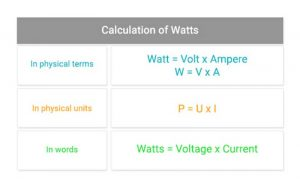### What are Wattages

Watts is basically made of voltages and amperages in combination. The calculating formula is easy to remember which is: Voltage x Amperage = Wattage. See the diagram for a visual demonstration. By doing this calculation, this will define the total amount of electricity that goes into an electrical system.

The Volts and the Amps work together to calculate what the total wattage rating will be. Let’s say we have two wires, one wire has high voltage or electrical pressure but the electrical wire is small, while the other wire has low electrical pressure, but it’s a very big electrical wire. The two wires differ in voltage or electrical pressure, but both electrical wires provide the same amount of wattage in the same amount of time or they produce the same wattage rating.

In electrical systems, some wires can produce high voltages with low amperages, while other wires transmit low voltages with high amperages. As an end result, the same amount of power or wattages gets produced by these two wires. For example, if we have a solar panel producing 15 Volts but only 3 Amps, this will mean, 15V x 3Amp = 45Watt. Let’s turn this around, if a solar panel produces 3 Volts and 15Amps, it will be: 3V x 15A = 45Watt. So basically, both solar panels produce the same amount of power, even though, they differ in Volt and Amp ratings. The only factor in this scenario is, that one solar panel will require a larger electrical cable than the other. Always remember, the larger your Amp rating is, the larger the electrical wire will need to be to transmit the amount of power. Let’s say you have a 200Watt solar panel at 20Volts, we need to determine the amount of amperage for the system. It is easy, here is the formula: 200Watt / 20V = 10Amps. This works the same for determining the Voltage if you have the Watt and amperage ratings. So as a conclusion, wattage can tell you the total amount of power that is generated or consumed by electrical appliances.

### What are Watt-hours

To determine your Watt-hours, you calculate your Wattage usage with time to determine how many watts or kilowatts per hour you generate or consume. A good example of this is, if you inspect your electricity bills, it can inform you about how many Watts (it will usually be in thousands) or more commonly, your kilowatts used over a period of time. This is a very basic calculation which is: Wattage x hours = Watt-hour rating. Let’s say you consume 400Watts in one hour, that will be 400-watt hours. (400Watt x 1hour).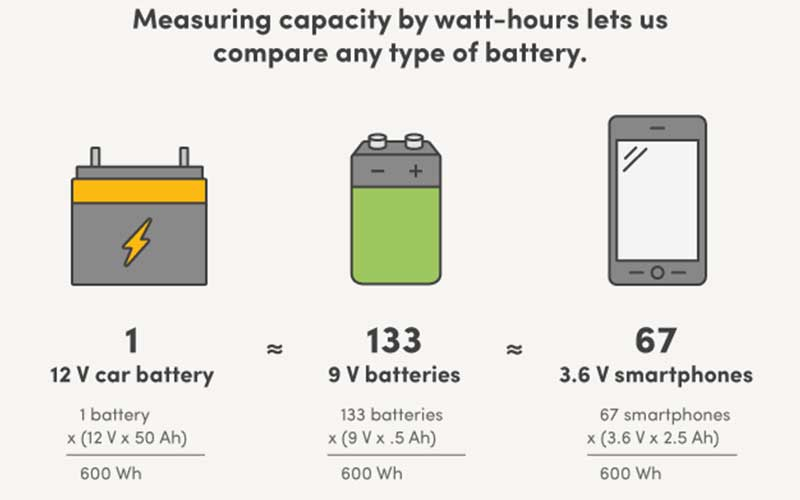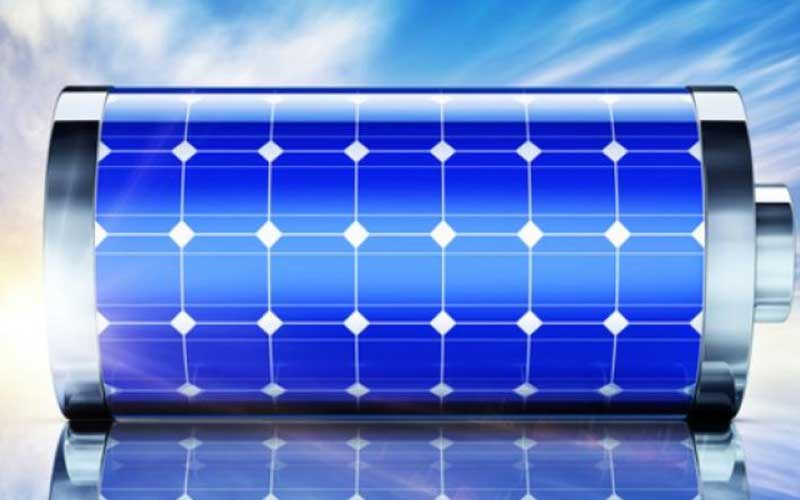### How much electricity can a battery store?

So, we know how to calculate electricity usage. Now, let’s take a look into calculating the amount of electricity that can be stored in a battery. If you have a battery, it will usually indicate a Voltage and Amperage rating. If your battery is 200Ah at 12V (the battery can produce 200amps at 12 volts for one hour), we need to determine the watt-hours first such as this: 200ah x 24V = 2400-watt hours. If you have the watt-hour rating for the battery, it will be the best way to compare batteries for your solar storage application. Take into consideration, the amp hours of a battery might be high but it comes at a specific Volt rating.

### Calculating how long a battery can provide power

Let’s take our 2400-Watt hour solar power storage battery. We want to power a 400-watt electrical load. The formula would be like this: 2400Wh / 400Watt load = 6 hours of power production. Generally speaking, a 2400wh solar battery can power a 400Watt load for 6 hours. As you can see, it is much easier to compare electrical devices for generating electricity or consuming electricity, by the means of using Watt-hours. We use volts and amps to determine the amount of wattage and then, using watts with time to determine what hours which we can use to determine how large a battery is, how long a battery will need to recharge and how long a battery can provide power for electrical appliances.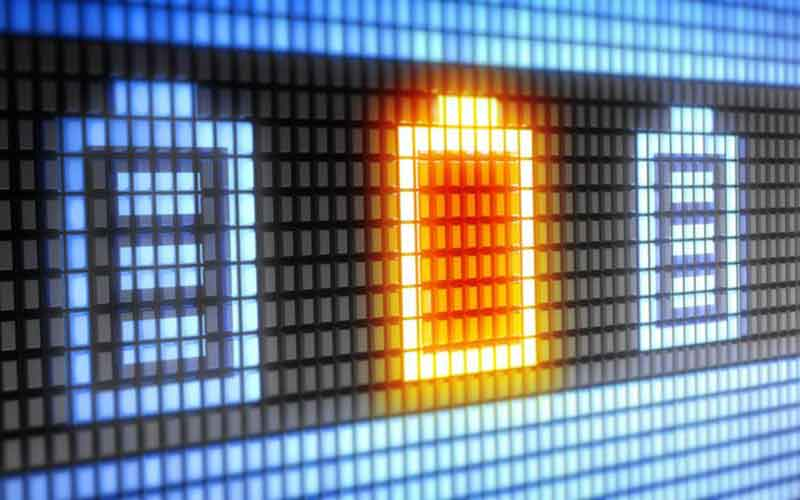### How to plan for your own solar system

To plan for your own solar system configuration, we will first look at the battery we are going to use.

Let’s say we use 2 x 12V 200Ah SLA batteries. If we configure these batteries in a parallel connection, we are going to multiply the Amp-hours by 2 batteries: 200Ah x 2 = 400Ah at 12 Volts. To determine the Watt-hours of these batteries, we will do the following: 400Ah x 12 Volts = 4800-watt hours. Because SLA or sealed lead-acid batteries have a DoD of 50%, we need to adjust the watt-hours to accommodate the DoD figure. Therefore: 4800Wh / 2 = 2400Wh. So, now we have to the wattage of our battery storage. Next, we need to receive the power to charge the batteries by means of solar electricity.

If we use a 300Watt solar panel at 32 Volts and 9.3 amps because of 32.5 V x 9.24 A = 300,3 Watts. So, if we install 2 x solar panels for our 2 x solar battery bank, it will be: 300,3 Watts x 2 = 600,6 Watts total energy from the solar panels.

### Calculating the size of charge controller required

To determine the size of the solar charge controller, we need to consider that solar charge controllers such as PWM’s and MPPT’s are rated by the means of Amperages. Therefore, we need to determine the number of amperages at 12Volts our 32.5V solar panel set will produce. So, we need to use the total watts from the solar panel array which is 600,6 Watts and divide it by 12 Volts: 600,6 Total watts / 12 Volts = 50 Amps. This means the amount of 50 Amps is what our PWM or MPPT device should be able to handle. However, the solar charge controller should not exactly match the amperages required, but, the solar charge controller should be slightly larger in Amps than the required amperages from the solar panels. We would recommend an MPPT solar charger such as the Epsolar eTracer 6415BND 150V/60A MPPT Charge Controller. An important note to remember regarding solar charge controllers is, when purchasing a solar charge controller, ensure that you measure and do your calculations based on the Amp output of the solar charge controller and not the input. Solar charge controllers are rated by the Amp output they offer and not by the Amp input.

### Solar battery charging duration

Now, we have a solar array producing electricity, we also have a battery bank storing electricity and lastly, we determined the size solar charge controller we require. We should now calculate the time duration for the battery bank to recharge. Our battery bank was rated at 2400Wh when we took our battery DoD into consideration. Our solar panel array is producing 600,6 Watts therefore: 2400Wh / 600,6 PV watts = 3,9 hours to recharge batteries. Many solar panels only output about 70% of their rated wattages, so to work in the 70% we will take the 600,6 Watts x 0.7 = 420,42 Watts. Therefore, to make a more accurate calculation, we take the 2400Wh / 420,42 Watts = 5,7 hours to recharge the batteries.

### Calculating battery bank runtime

We have a 2400Wh battery bank and we want to power a 100Watt load. We will need to the following calculation: 2400Wh / 100Watt load = 24 hours of runtime. However, this is if the battery is fully charged. If way takes a more realistic load such as 700 watts, we will only get 3,4 hours of runtime.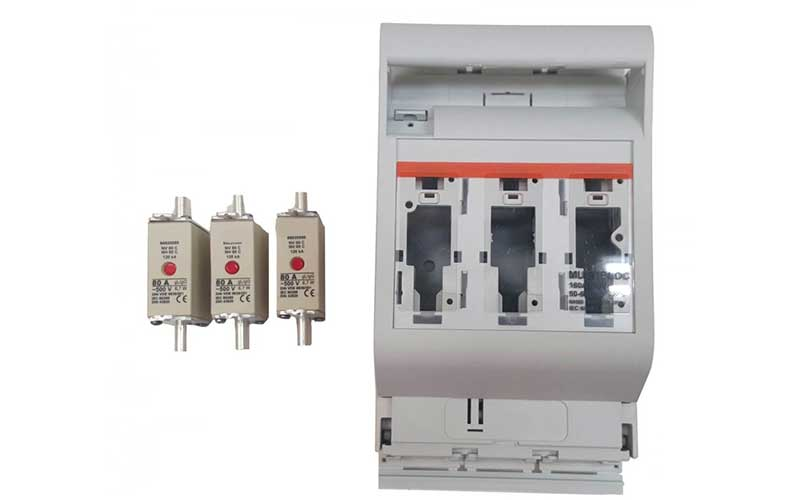### Electrical protection fuses

What is an electrical protection fuse? A fuse is a small conductor that heats up when a specific amp rating is met. If a large number of amps reach a fuse, it will disconnect the electrical component or the electrical wire. If you’re connecting your electrical devices to a battery, the battery will generate large amounts of amps, therefore, protection against all the amps is necessary. This is why sizing a solar configuration system sizing is important. If you use the wrong wires and do not add the necessary protection devices, the large amount of DC electricity can lead to heat in wires and can result in fires.